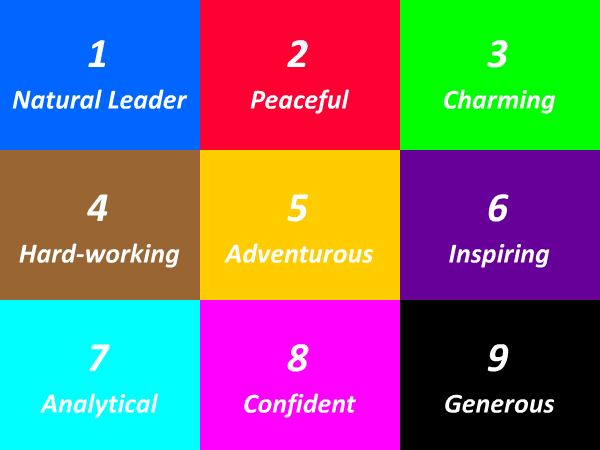# 61 Times Table - Generate Multiplication Table of 61

61 Times Table
1x61=61
2x61=122
3x61=183
4x61=244
5x61=305
6x61=366
7x61=427
8x61=488
9x61=549
10x61=610
11x61=671
12x61=732
13x61=793
14x61=854
15x61=915
16x61=976
17x61=1037
18x61=1098
19x61=1159
20x61=1220
21x61=1281
22x61=1342
23x61=1403
24x61=1464
25x61=1525
61 Times Table
26x61=1586
27x61=1647
28x61=1708
29x61=1769
30x61=1830
31x61=1891
32x61=1952
33x61=2013
34x61=2074
35x61=2135
36x61=2196
37x61=2257
38x61=2318
39x61=2379
40x61=2440
41x61=2501
42x61=2562
43x61=2623
44x61=2684
45x61=2745
46x61=2806
47x61=2867
48x61=2928
49x61=2989
50x61=3050
61 Times Table
51x61=3111
52x61=3172
53x61=3233
54x61=3294
55x61=3355
56x61=3416
57x61=3477
58x61=3538
59x61=3599
60x61=3660
61x61=3721
62x61=3782
63x61=3843
64x61=3904
65x61=3965
66x61=4026
67x61=4087
68x61=4148
69x61=4209
70x61=4270
71x61=4331
72x61=4392
73x61=4453
74x61=4514
75x61=4575

## Test Your Skills Here

The 61 times table quiz is very useful in the improvement of kid's multiplication and maths skills. Here you will find a selection of times table tests designed to help your child to learn and practice their times tables. Fill in your answers. Once you have entered all the answers, click on 'Check' button to see whether you have got them all correct. If your answers is Wrong then we will show you the 'Correct' answer.

Practice Excersise
61 x = 1098
61 x = 1403
61 x 20 =
61 x 29 =
61 x = 1647
61 x = 1037
61 x 4 =
61 x = 732
61 x 25 =
61 x 23 =#### More Tables#### Top Search Quesries for this page:

• 61 times table
• 61 times tables
• table of 61
• 61 multiplication table
• multiplication chart 61
• multiplication of 61
• 61 table maths### Spreading Knowledge Across the World

USA - United States of America  Canada  United Kingdom  Australia  New Zealand  South America  Brazil  Portugal  Netherland  South Africa  Ethiopia  Zambia  Singapore  Malaysia  India  China  UAE - Saudi Arabia  Qatar  Oman  Kuwait  Bahrain  Dubai  Israil  England  Scotland  Norway  Ireland  Denmark  France  Spain  Poland  and  many more....